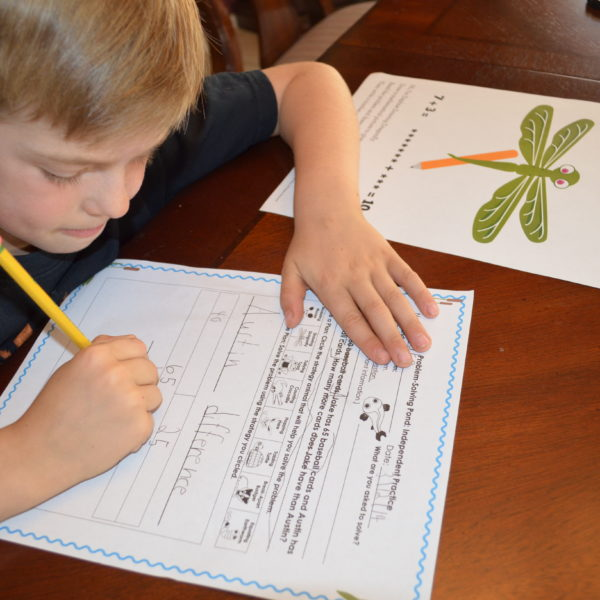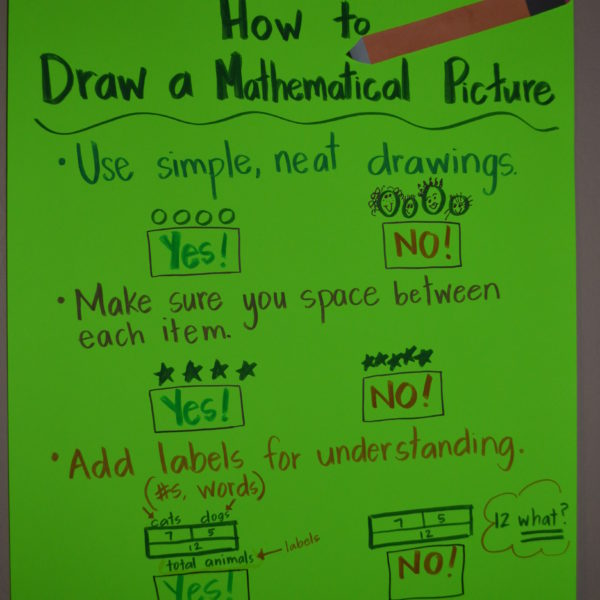### Introducing Daphne Drawing Dragonfly

Hi, I’m Daphne the Drawing Dragonfly.
Draw a mathematical picture to give my strategy a try.
Read the problem and draw what’s going on;
Then write a number sentence for what you have drawn.

Daphne Drawing Dragonfly is our newest animal in our Problem-Solving Pond: A Common Core Math Strategy Unit. The Problem-Solving Pond was created to help teachers overcome Common Core math challenges and employ problem-solving strategies with confidence and fidelity. Daphne’s unit is perfect for general education, special education, RTI and math intervention. Read more about Daphne’s strategy below or download the complete unit here.

WHAT is drawing? Students make a visual representation of the story problem such as a picture, bar model, tens frame or array.WHY is drawing important? When students use the drawing strategy, they are making a concrete representation, strengthening understanding of the mathematical concepts.  Effective math classrooms include frequent use of pictorial representations to help students process and visualize mathematical concepts learned.

HOW do I teach drawing?Teach students to create neat, organized drawings with labels and numbers. Students will need to be taught the bar model and arrays, but it is best to let students create the pictorial representation that they see and works for them.

WHEN should I use drawing? This strategy is ideal for presenting a new mathematical operation.  Most teachers use this strategy with K-2 mathematicians, but this is also beneficial for older students as it works especially well with money, fractions, ratios and percentages. Drawing is a great way to double-check solutions because the visual representation increases understanding of the problem.

• Create drawing guidelines. As a class, decide important components to include in a mathematical drawing. Possible components include:
• Simple drawings
• Appropriate spacing
• Neat labels (words or numbers)